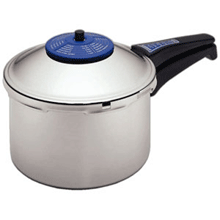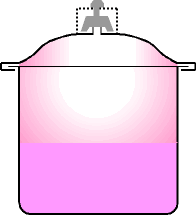Ch 1. Basics Multimedia Engineering Thermodynamics System Temperature& Pressure Heat andWork Energy
 Chapter 1. Basics 2. Pure Substances 3. First Law 4. Energy Analysis 5. Second Law 6. Entropy 7. Exergy Analysis 8. Gas Power Cyc 9. Brayton Cycle 10. Rankine Cycle Appendix Basic Math Units Thermo Tables Search eBooks Dynamics Fluids Math Mechanics Statics Thermodynamics Author(s): Meirong Huang Kurt Gramoll ©Kurt GramollTHERMODYNAMICS - CASE STUDY IntroductionPressure Cooker Jack's mom bought a new pressure cooker. Jack is interested in the petcock, which is a small piece of mass, sits on top of the only opening in the middle of the lid and prevents steam from escaping until the pressure force overcomes the weight of the petcock. He just wonders the weight of the petcock which can maintain a high pressure inside the cooker. What is known: The operation pressure is 100 kPa gage The opening cross-sectional area is 4 mm2 Atmospheric pressure is 101 kPa QuestionsPressure Cooker What is the mass of the petcock? Approach Solve the problem using the basic steps in engineering problem solving: 1) read 2) draw diagrams 3) write equations 4) solve and 5) check solution. For step 3, use the force balance in the base of the petcock: F - ( Fa+ G ) = 0